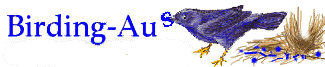birding-aus [Top] [All Lists]

## Nick Lesenburg's e-mail

 To: Nick Lesenburg's e-mail John Leonard <> Tue, 15 Feb 2000 08:33:05 +1100
 ```Does anybody have Nick Lesenburg's e-mail; he doesn't appear to be resubscribed. Thanks John Leonard ^^^^^^^^^^^^^^^^^^^^^^^^^^^^^^^^^^^^^^^^^^^^^^^^^^^^^^^^^^^^^^ John Leonard (Dr), PO Box 243, Woden, ACT 2606, Australia m("spirit.com.au","jleonard"); http://www.spirit.net.au/~jleonard ^^^^^^^^^^^^^^^^^^^^^^^^^^^^^^^^^^^^^^^^^^^^^^^^^^^^^^^^^^^^^^ To unsubscribe from this list, please send a message to m("vicnet.net.au","majordomo"); Include ONLY "unsubscribe birding-aus" in the message body (without the quotes) ```
 Current Thread Nick Lesenburg's e-mail, John Leonard <=